﻿ Thermodynamic and Surface Properties of Cr-X, (X = Mo, Fe) Liquid AlloysAmerican Journal of Condensed Matter Physics

p-ISSN: 2163-1115    e-ISSN: 2163-1123

2017;  7(3): 57-66

doi:10.5923/j.ajcmp.20170703.01### Thermodynamic and Surface Properties of Cr-X, (X = Mo, Fe) Liquid Alloys

Y. A. Odusote, A. I. Popoola

Department of Physics, The Federal University of Technology, Akure, Nigeria

Correspondence to: Y. A. Odusote, Department of Physics, The Federal University of Technology, Akure, Nigeria.
 Email: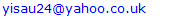Abstract

Thermodynamic and surface properties of Cr-Mo and Cr-Fe liquid alloys were studied using a simple scheme based on the formation of self-associates in conjunction with a statistical model founded on the concept of the layered structure near the interface. The phase-segregation tendencies in these liquid alloys were analyzed through the study of surface properties (surface tension and surface composition), transport properties (diﬀusion and viscosity) and microscopic functions (concentration ﬂuctuations in the long-wavelength limit, Scc(0) and chemical short-range order parameter, α1). The positive deviation of the properties of mixing of the two liquid alloys from ideality has been discussed. Results show that the degree of phase-separation in Cr-Fe, as gauged by the Scc(0) and α1, is less than in Cr-Mo. The theoretical analysis reveals that the rate at which Cr-atoms segregate at the surface is higher in Cr-Fe, than in Cr-Mo alloys for all bulk concentrations.

Keywords: Modelling, Segregation, Cr-Mo, Cr-Fe, Thermophysical properties

Cite this paper: Y. A. Odusote, A. I. Popoola, Thermodynamic and Surface Properties of Cr-X, (X = Mo, Fe) Liquid Alloys, American Journal of Condensed Matter Physics, Vol. 7 No. 3, 2017, pp. 57-66. doi: 10.5923/j.ajcmp.20170703.01.

### 1. Introduction

Metal alloys in general oﬀer some beneﬁts over convention metals due to their increased mechanical strength, heat and chemical resistance, and reduced production costs. From technological viewpoints, binary and ternary alloy systems containing chromium such as Cr-Mo, Cr-Fe, Cr-Al, Cr-Mo-Al, Cr-Nb-Re and Cr-Fe-Mo-C etc., represent a class of alloy systems with excellent high-temperature strength, high ductility, good corrosion and oxidation resistance. These properties make them sought after in a wide range of applications from steel production and Ni-based super alloys to surgical implants [1, 2], including aerospace and automobile industries , structural materials for nuclear power plants [1, 4], complex bulk metallic glasses , heat-resistant and corrosion-resistant protective coatings [2, 6].
In order to enhance the tribological, structural and mechanical properties of chromium-based alloys to suit diverse service conditions, alloying with other elements such as molybdenum (Mo) has shown to be eﬀective in improving the toughness and tribological properties of Cr-N coatings [7-11]. On the other hand, the ability of Mo to form Magnli phase oxides can be used to prepare materials with solid lubricant potential, which considerably reduce the coeﬃcient of friction [12, 13].
The present work is motivated by the controversial and divergent results in literature concerning chromium-based alloys. For instance, thermodynamic measurement performed on solid Cr-Mo alloys in the temperature range of 1471 - 1773 K [14-17] showed that the data of Dickson et al.  are at variance with those of others. While negative deviations from ideality was reported for components activity coeﬃcients of Cr-Mo alloys at 1673 K using the Knudsen eﬀusion technique by Dickson et al. . The measured activity of Cr in solid Cr-Mo alloys at 1873 K by Jacob and Kumar , the results of Laﬃtte and Kubaschewskil , and that of Kubaschewski and Chart  on the other hand, indicated positive deviations from ideality. Similarly, Cr-Fe has been studied widely both experimentally and theoretically by many authors [1, 18-27]. However, a recent review by Xiong et al. [26, 27] indicated that the commonly accepted result due to Andersson and Sundman  requires further thermodynamic reassessment in many aspects, among which is precise locations of the corresponding phase boundaries of the solidus and liquidus lines on its phase diagram .
At low temperatures, Cr-Mo and Cr-Fe alloys are endowed with miscibility gap of the body centered cubic (bcc) in the solid phase. Alloys within the miscibility gap tend to separate or decompose into a Cr-rich bcc phase (α’), Mo-rich bcc phase (α) and/or an Fe-rich bcc phase (α) [27, 28]. This phenomenon of phase separation in Cr-based alloys, known generally as the “475° embrittlement” has resulted in increased hardness and reduced ductility in these alloys with aging [20, 28, 29]. Also, Quintela et al.  recently observed that even a very small addition of only ≈ 1% of Mo or W to Cr-based coating alloys, lead to a spontaneous phase segregation. Consequently, attempts are being made by researchers to combine all available experimental information to achieve a consistent description of the phase equilibria and thermodynamics of the phases involved in Cr-Mo and Cr-Fe alloy systems [27, 31, 32].
The amount of research on Cr-based (both binary and higher order alloys in recent times, has being on steady increase due to its various industrial applications and the use of atomistic approaches in investigating their structural behaviour [1, 27, 28]. However, measurement of thermodynamic and thermophysical quantities of alloy systems containing elements such as Cr, which is a strongly electropositive metal like Mg, and often acts as a strong reducing agent are difficult. In particular, concerning the data of transport and surface properties of ternary Cr-based and its binary alloys, only the surface tension data of their pure components have been well documented . Since, a systematic measure of both thermodynamic and thermophysical data over the whole range of composition and temperature can be almost impossible to perform. It is therefore expected that most of the missing thermodynamic value on binary and multi-component systems will come within theoretical framework, rather than from direct experiment.
For the design and development of reliable materials for high-temperature applications, the knowledge of thermodynamic and thermophysical quantities of these alloys is important in gaining qualitative insight into their mixing behaviour as well as aid in elucidating the energetics and structure at atomistic level in the melts and how they are coupled. The nature of the atomic interactions and structural re-adjustment of the constituent atoms in liquid Cr-Mo and Cr-Fe alloys can be gauged from the values of Hume-Rothery empirical factors such as electronegativity diﬀerence (ECr-EMo = -0.20, and ECr-EFe = -0.20) , the size ratio, VMo/VCr ≈ 1.30 and VFe/VCr ≈ 0.98 , and valency diﬀerence (=0 or =2) , respectively. Obviously, these factors can only provide partial information and are not suﬃcient to completely characterize the mixing behaviour in these alloys.
In the present study, a simple scheme developed by Singh and Sommer  for studying liquid alloy systems with miscibility gap tendencies has been utilized to model liquid Cr-Mo and Cr-Fe alloys at 1471 K and 1600 K, respectively. We intend to explain the energetic eﬀects on these systems in terms of the interaction energy parameter W, that reproduces as closely as possible their various concentration dependent properties with a view to understand alloying nature of ordering or segregation tendencies in the molten alloys. Afterwards, the transport properties (diﬀusion and viscosity), microscopic functions (concentration-concentration ﬂuctuations in the long-wavelength limits, Scc(0) and chemical short-range order parameter, α1) and surface properties (surface concentration and surface tension) were studied from a theoretical viewpoint with the aim of correlating the properties of the liquid alloys in the bulk and at the surface.
Both Cr-Mo and Cr-Fe liquid alloys exhibited positive deviations from ideal mixture behaviour with positive energy parameters, W at the respective temperatures. The positive signs of energy parameters for Cr-Mo and Cr-Fe alloys imply repulsive interactions between constituents of the alloys, and further indicate that there is a general tendency for homo-coordination of atoms in both liquid alloy systems .
The theoretical formulations of the model based on the formation of self-associates in binary liquid alloys as related to the various thermodynamic quantities, microscopic functions, transport and surface properties are presented in the next section. This is followed by results and discussion in section 3, and conclusion in the last section.

### 2. Theoretical Formulation

Let a liquid binary alloy consists of NA = NcA atoms of element A and NB = NcB atoms of element B located at equivalent lattice sites, where the total number of atoms N = NA + NB and form of a polyatomic matrix, leading to the formation of like-atom cluster or self-associates of the type Aµ and Bν, i.e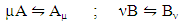(1)
µ and ν are the numbers of atoms in the cluster of Ai and Bj type, and ci, (i = A, B) being the mole fraction of components A and B in the alloy, respectively.

#### 2.1. Thermodynamic and Microscopic Functions for the Bulk

Based on the assumption of self-associates, the standard expression for the Gibbs free energy of mixing, GM for demixing liquid binary alloys which is dependent on the number of self-associates, n = µ/ν can be expressed as :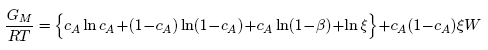(2)
with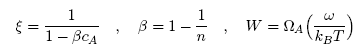(3)
and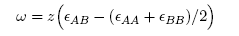(4)
The coordination number of the liquid alloys z is taken as 10 [37-39]. ϵij are the energies of i−j pairs of atoms and ω deﬁned by Eq. (4) is known as the interchange energy whose value gives insight into the nature of alloying in binary liquid alloys. Clearly, for a liquid alloy if ω < 0, there is tendency to form unlike atom pairs and if it is greater than zero, like atoms tend to pair together as nearest-neighbors. ω = 0, indicates that atoms in the mixture are perfectly disordered .
The concentration-concentration ﬂuctuations at the long wavelength limits, Scc(0) can be readily obtained using standard thermodynamic relation in terms of the free energy of mixing as [36-39]: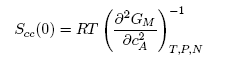(5)
Using Eqns. (2) and (5), an expression for Scc(0) is given by: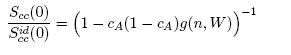(6)
where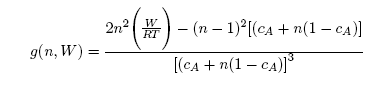(7)
for ideal mixing, the energy parameter ω in Eq.(4) equal to zero, and Eq.(6) reduces to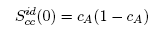(8)
In the present approach, n and W are independent of concentration but may depend on pressure and temperature. The parameters (n, W) are the model parameters to be ﬁtted at a given temperature to calculate the thermodynamic, transport and by extension the structural properties of the binary liquid alloys.
The Warren-Cowley chemical short range order parameter, (α1), [40, 41] has been used to quantify the degree of order and segregation in the liquid alloys. This parameter α1 is computed theoretically [36-38] via the relation: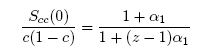(9)
In similar fashion with the aid of Eq. (2), expressions can be obtained for other thermodynamic quantities via standard thermodynamic relations: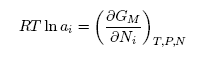(10)
solving Eq. (10) and recall that N = NA +NB with cA = NA/N, one obtains expressions for the component activities as: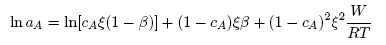(11)
and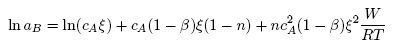(12)
The enthalpy of mixing HM is given as: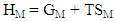(13)
and the entropy of mixing, SM is deﬁned in terms of GM as: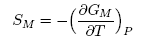(14)
putting Eq. (2) in Eq. (14), the entropy of mixing becomes: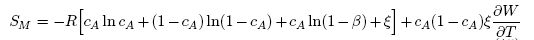(15)
Segregating alloy systems are endowed with positive values of HM.

#### 2.2. Transport Properties: Diﬀusion and Viscosity

##### 2.2.1. Diﬀusion
The formalism that relates diﬀusion and Scc(0) combines Darken’s thermodynamic equation for diffusion with basic thermodynamic equation in the form [36, 39]: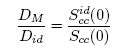(16)
where DM is the mutual diﬀusion coeﬃcient and Did is the intrinsic diﬀusion coeﬃcient for an ideal mixture. An understanding of Eq. (16) shows that DM/Did < 1 and DM/Did > 1 are signatures of homocoordination and heterocoordination, respectively. In addition, if DM/Did → 1, it implies ideal mixing .
##### 2.2.2. Viscosity
Taking into account the Strokes-Einstein type relation for liquid alloys, the viscosity, η is related to the Scc(0) and DM (Eq.(16), by [42-43]: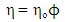(17)
with the quantities ϕ and ηo deﬁned as: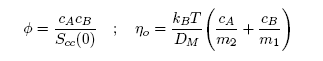(18)
m1 and m2 are functions of the size and shape of the constituent particles; kB is the Boltzmann’s constant and T is the absolute temperature.
The thermodynamic relevance of the quantity ϕ has been well reported for a number of binary liquid alloys [36, 38, 43]. The expression of ϕ that incorporates both the entropic (via the number of self-associates, n) and the enthalpic contributions (via the interaction energy parameter, W) is given  as: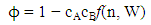(19)
Taking the last term of Eq. (3) with Eqs. (7), (17) and (19), one obtains the expression for viscosity in terms of the entropy and enthalpy as in :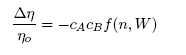(20)
where ∆η = η−ηo is the deviation in η.
The factor f(n, W) is responsible for the characteristic behavior of viscosity for a given binary alloy. In the light of Conformal solution [42, 44, 45], another expression for viscosity is written in terms of the enthalpy of mixing, HM as: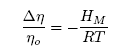(21)
R is the universal gas constant.

#### 2.3. Surface Properties: Surface Concentration and Surface Tension

The grand partition functions set up for the surface layer and that of the bulk provides a link between the bulk and the surface properties within the frame of the statistical mechanical approach through the concept of layered structure near the interface [46, 47]. This linkage connects the surface,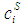and bulk,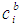concentrations in terms of surface tension, σ via a pair of net equation: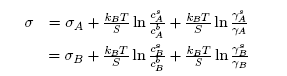(22)
where σA and σB are the surface tensions of the components A and B, respectively. S is the mean atomic surface area of the alloy, calculated using the relation :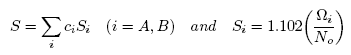(23)
i is the atomic volume and No is the Avogadro’s number.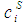and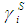refer to the concentration and activity coeﬃcient of the ith component at the surface, respectively.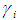and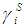are related by: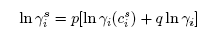(24)
here p and q are the surface coordination fractions deﬁned as the fractions of the total number of nearest neighbours of an atom in its own layer and that in the adjoining layer. As a consequence, p + 2q = 1. For a closed-packed structure the values of these parameters usually are taken as p = 0.5 and q = 0.25, respectively .

### 3. Results and Discussion

The thermodynamic data used for the modelling of the bulk thermodynamic properties of liquid Cr-Mo and Cr-Fe alloys at 1471 K and 1600 K, respectively, were taken from [14, 22]. The basic inputs for the computation of the bulk properties are the interaction energy parameter, W, its temperature dependence ∂W/∂T, and the number of self-associates, n, all listed in Table 1 for each of the two liquid alloys investigated. The values of these parameters (W, ∂W/∂T and n) were adjusted by the method of successive approximation to give the concentration dependence of activity, ai, GM/RT, HM /RT, SM/R and Scc(0) that reproduces fairly well the corresponding thermodynamic data as shown in Figs. 1 to 6. The interaction energy parameters for liquid Cr-Mo and Cr-Fe alloys, expressed in RT units, are W = 1.6200 and 0.7100, in that order, and remain invariant in all calculations.
 Table 1. Energy parameters used for the bulk properties calculations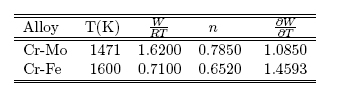#### 3.1. Thermodynamic Properties: ai, GM, HM and SM

Figs. 1 and 2 show the plot of the calculated component activities of each liquid alloys studied compared with the respective experimental data. In Fig. 1, activity of Cr was compared at two diﬀerent temperatures 1471 K  and 1873 K  for which experimental values exists in the literature. It can be noticed that there are fairly reasonable agreement between the calculated and experimental data especially below 0.7 atomic faction of Mo in Cr-Mo at 1471 K, while aCr in Cr-Mo has better agreement below 0.5atomic faction at 1471 K and also at cCr > 0.5 for aCr at 1873 K in Cr-Mo liquid alloys (Fig. 1). Above these concentrations, the calculated values show some form of disagreement with experimental data. Since activity is one of the thermodynamic functions that can be measured directly from experiment, the inconsistencies observed could suggest further assessment of thermodynamic activity data of Cr-Mo liquid alloys. On the other hand, Fig. 2 shows excellent agreement with experimental data of component activities of Cr and Fe in Cr-Fe liquid alloys at 1600 K, with the activity values very close to ideal behaviour for aCr and aFe, respectively, [1, 14].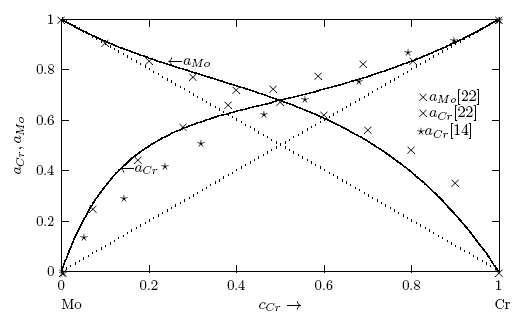Figure 1. Concentration dependence of component activities aCr and aMo in Cr-Mo liquid alloys computed using Eqs. (11) and (12) at 1471 K, respectively. ⋆ ⋆ . . .and x x. . . are measured activity data at 1873 K and 1471 K, respectively. The solid lines denote calculated values. cCr is the bulk concentration of Cr in the alloy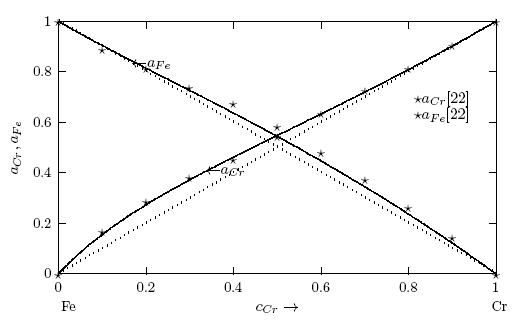Figure 2. Concentration dependence of component activities aCr and aFe in Cr-Fe liquid alloys computed using Eqs. (11) and (12) at 1600 K, respectively. Symbol ⋆⋆. . . measured activity data at 1471 K. The solid lines denot calculated values. cCr is the bulk concentration of Cr in the alloy
The concentration dependence of GM/RT computed using Eq. (2) for the two liquid alloys are presented in Fig. 3 with the respective experimental data, including the symmetry of the curves describing the GM/RT vs. ccr at the equiatomic composition. The degree of compound formation tendency in liquid alloys can be gauged by the normalized form of the Gibbs free energy of mixing of liquid phase at the equiatomic composition. The values of GM/RT between 0 and -1 are characteristic for weakly interacting systems, those between -1 and -2 indicate systems with moderate interactions, while the values lower than -3 are typical of strongly interaction systems [38, 49, 50]. From Fig. 3, it is observed that the curves describing the GM/RT show excellent agreement between calculated and experimental values across the whole concentration range with Cr-Mo and Cr-Fe liquid alloys exhibiting minimum GM values of -0.3419 RT and -0.5773 RT at cCr = 0.5, respectively, indicating that the tendency of compound formation in the liquid phase of these systems is weak in nature. Also from Fig. 3, one can deduce that the degree of segregation in Cr-Mo with (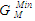= -0.3419 RT) is higher than in Cr-Fe with (= -0.5773 RT). The excellent agreement between the two sets of data also suggests that the choice of n and W used for the model calculations is quite reasonable.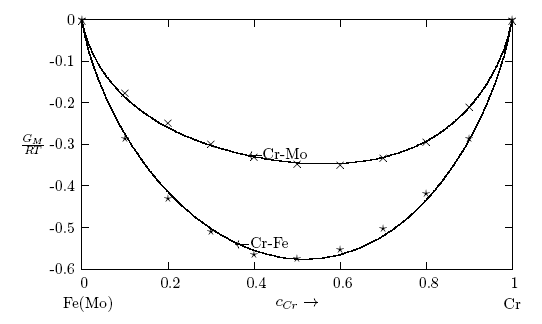Figure 3. Concentration dependence of free energy of mixing, GM/RT of Cr-Fe and Cr-Fe liquid alloys at 1471 K and 1600 K, respectively. The solid line denotes theoretical values, while times and stars denote experimental data  for Cr-Mo and Cr-Fe at respective temperatures. cCr is the bulk concentration of Cr in the alloy
The enthalpy of mixing, HM and the entropy of mixing, SM have been calculated from Eqs.(13) and (15), in that other, using the same interaction parameters given in Table 1 as used in computing ai and GM. The plots of HM/RT and SM/R versus cCr for the two Cr-based liquid alloys investigated at 1471 K and 1600 K are depicted respectively in Figs. 4 and 5, which show that like the experimental values , both HM and SM are positive at entire concentrations of Cr. There exists a reasonable agreement between calculated and experimental values.
The values of maxima in HM/RT (Fig. 4) are observed around cCr = 0.46 with HM/RT = 0.5965 and 0.4338 for Cr-Mo and Cr-Fe liquid alloys respectively, corresponding to stoichiometric concentration about the equiatomic composition. It is interesting to observe that the isotherms describing both the calculated and experimental values of the HM/RT and SM/R for the two liquid alloys are symmetric with respect to the equiatomic composition (typical of segregating alloys) . The SM/R shows maxima values of 0.9373 and 1.0042 at cCr = 0.5 for Cr-Mo and Cr-Fe liquid alloys, respectively (Fig. 5). We observed during the computation that if the interaction energy parameters are assumed to be temperature independent, (i.e. if ∂W/∂T = 0), both HM/RT and SM/R so obtained were very inconsistent with experimental values. The theoretical analysis reveals that the interaction energy parameter, W is temperature dependent.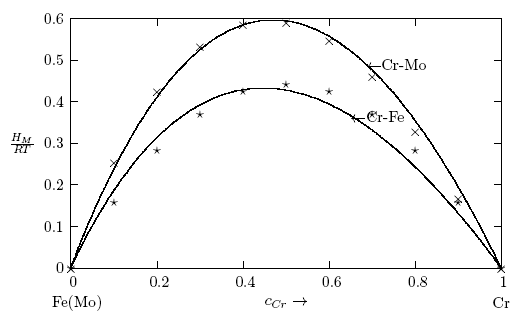Figure 4. Concentration dependence of enthalpy of mixing, HM/RT of Cr-Fe and Cr-Fe liquid alloys at 1471 K and 1600 K, respectively. The solid line denotes theoretical values, while times and stars denote experimental data  for Cr-Mo and Cr-Fe at respective temperatures. cCr is the bulk concentration of Cr in the alloy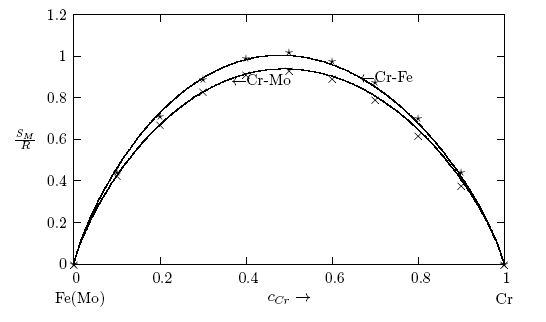Figure 5. Concentration dependence of entropy of mixing, SM/R of Cr-Fe and Cr-Fe liquid alloys at 1471 K and 1600 K, respectively. The solid line denotes theoretical values, while times and stars denote experimental data  for Cr-Mo and Cr-Fe at respective temperatures. cCr is the bulk concentration of Cr in the alloy

#### 3.2. Microscopic Functions: Scc(0) and α1

The concentration-concentration ﬂuctuations in long-wavelength limits, Scc(0) has emerged as a very useful thermodynamic function to investigate the atomic order in a binary liquid alloy. The deviation of Scc(0) from the ideal values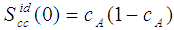(Eq. 8) can be used as a measure of the nature of atomic order and the stability of the mixture at a given composition. If at a given composition,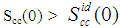, there is a tendency of segregation. On the contrary,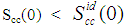refers to hetero-coordination. The theoretical values of Scc(0) are computed via Eq.(6) using the same interaction parameters used to compute GM. The measured Scc(0) were determined from the experimental Gibbs free energy of mixing data taken from  when Eq.(5) is solved numerically. The results are shown in Fig. 6. From the ﬁgure, it is evident that the computed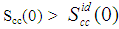across the entire concentration range of Cr for both Cr-Mo and Cr-Fe liquid alloys at 1471 K and 1600 K, respectively. This suggests that both Cr-Mo and Cr-Fe are segregating liquid alloys. The degree of deviation observed around the equiatomic composition in Cr-Mo is about 62% higher than that of Cr-Fe. That is to say that homo-coordination of atoms (preference for like atoms to be paired as nearest-neighbours) exists in these molten alloy systems, which is consistent with positive values of the interaction energy parameter, W. Also from Table 1, it can be observed that when n and W are less than 1 for Cr-Fe alloys, the Scc(0) exhibits symmetrical behaviour at equiatomic composition with cCr = 1/2, whereas, Cr-Mo liquid alloys with n < 1 and W > 1, exhibits asymmetry and positive deviation in Scc(0) as expected as a function of both n and W. However, the positive deviation observed in Scc(0) behaviour for Cr-Fe liquid alloys at 1600 K in this work contrast sharply with the results of Novakovic and Brillo  who used the quasi-chemical approximation for regular alloys and reported that Cr-Fe alloys exhibited negative deviation with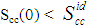at 1873 K.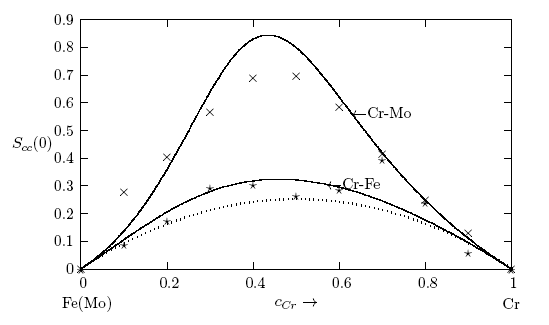Figure 6. Concentration-concentration fluctuations at long wavelength limits, Scc(0) as a function of concentration for Cr-Mo and Cr-Fe liquid alloys at 1471 K and 1600 K, respectively. The solid line denotes theoretical values, while times and stars denote experimental data  for Cr-Mo and Cr-Fe at respective temperatures. The dots denote the ideal values of Scc(0). cCr is the bulk concentration of Cr in the alloy
In order to gain qualitative insight into the nature of arrangement of atoms, the Warren-Cowley short range order parameter, α1, was also computed for both liquid alloys studied. This parameter (α1) can be evaluated theoretically using the knowledge of Scc(0) through Eq.(9). At equiatomic composition, one has -1≤ α1 ≤ +1. The minimum value of α1 = -1 implies complete ordering, α1 = +1 corresponds to segregation leading to the phase separation and α1 = 0 implies a random distribution of atoms. It is found from Fig. 7 that α1 is positive at all concentrations of Cr in Cr-Mo and Cr-Fe liquid alloys which is indicative of segregation or phase separation as evident from Scc(0). In the present work, the value of α1 has been computed with the coordination number, z = 10, as shown in Fig. 7. From the ﬁgure, the asymmetry in α1 is distinctly visible at about cCr = 0.4. This further conﬁrms earlier assertion that both Cr-Mo and Cr-Fe liquid alloys are segregating systems of like atoms (i.e. Cr-Cr, Mo-Mo or Fe-Fe) pairing as nearest-neighbours.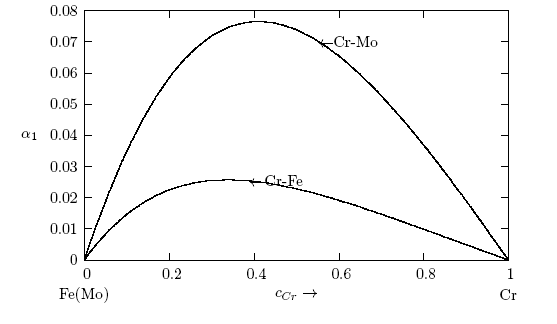Figure 7. Warren-Coyley Short-range order parameter, (α1), computed using Eq. (9) versus concentration for Cr-Mo and Cr-Fe liqud alloys at 1471 K and 1600 K, respectively. cCr is the bulk concentration of Cr in the alloys

#### 3.3. Diﬀusion and Viscosity

The calculated values of Scc(0) are used in Eq.(16) to assess the ratio of the mutual and self-diﬀusion coeﬃcients, DM/Did. The values of DM/Did are found to be less than 1 across the concentration range as presented in Fig. 8. This also indicates that the segregating feature of Cr-Mo and Cr-Fe liquid alloy as observed in Scc(0) and α1. Since DM/Did sharply reduced to very small values in the region around cCr ≈ 0.41 and 0.34 with distinct asymmetric features, where Cr-Mo and Cr-Fe liquid alloys have their minima values, respectively. The phase separating tendency in Cr-Mo and Cr-Fe liquid alloys at 1471 K and 1600 K is maximum at the intermediate composition.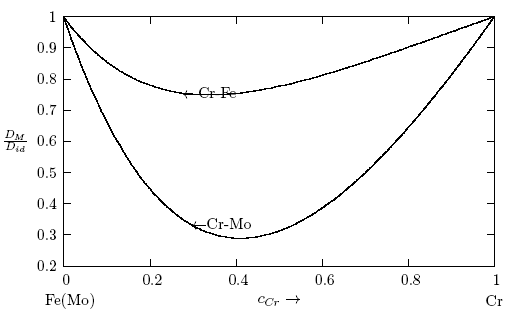Figure 8. Diffusion coefficients, DM/Did, computed using Eq.(16) versus concentration for Cr-Mo and Cr-Fe liquid alloys at 1471 K and 1600 K, respectively. cCr is the bulk concentration in the alloys
Owing to chemical reactivity and aﬃnity for oxygen by liquid metallic elements at elevated temperatures, experimental determination of viscosity data is usually very diﬃcult . This accounts for why a large missing gap exists between diﬀerent sets of experimental data or a nearly complete lack of viscosity data for many liquid alloys in literature. To overcome this challenges, many theoretical models have been proposed for estimating viscosity [42-43, 51-56], but none of the existing models is universally applicable to estimate viscosity data for a large class of liquid alloy systems. In this work, the expressions of viscosity due to Singh and Sommer  through Eqs. (20) and (21) have been used to model the viscosity of each liquid alloy. The negative values of experimental enthalpy of mixing calculated using Eq. (21) for Cr-Mo and Cr-Fe liquid alloys are the corresponding experimental values of viscosity for the alloys.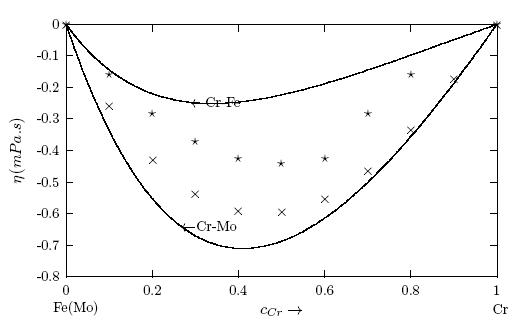Figure 9. Concentration dependence of viscosity, η for Cr-Mo and Cr-Fe liquid alloys calculated using Eq. (20) at 1471 K and 1600 K, respectively. The solid line denotes theoretical values, while times and stars denote –HM/RT used as experimental data  via Eq. (21) for Cr-Mo and Cr-Fe liquid alloys at respective temperatures
To the best of authors’ knowledge, the viscosity η of Cr-Mo liquid alloys has not yet experimentally been determined, while very old scatter data exists for Cr-Fe liquid alloys in literature [1, 57]. Fig. 9 shows the plot of the concentration dependence of viscosity for each liquid alloy at respective investigated temperatures. From the ﬁgure, it is seen that both the calculated and experimental viscosity isotherms for each liquid alloys show the same trend of negative deviation from ideality with asymmetric behaviour as observed in α1 and DM/Did. As a rule, the compound forming systems are apt to exhibit maximum (due to positive deviations) in their isothermal viscosity [38, 46] in those composition ranges where intermetallic compounds are formed in the solid state. One would therefore expect for segregating alloys like Cr-Mo and Cr-Fe with positive HM, to exhibit negative deviations of isothermal viscosity [36, 38, 58] as seen in Fig. 9 with positive values of W as well. The correlation between the calculated and experimental viscosity data shows a better agreement in Cr-Mo alloys than that of Cr-Fe liquid alloys.

#### 3.4. Surface Properties

Using the parameters given in Tables 1 and 2 as input and the required activities as obtained from the relevant expressions in the model, the surface concentration of Cr has been computed by solving Eq. (22) simultaneously. These surface concentrations were used to evaluate the surface tension as a function of bulk concentration, ci (i = Cr) for Cr-Mo and Cr-Fe liquid alloy at the working temperatures.
 Table 2. Essential parameters used for the surface properties calculation for Cr-Mo and Co-Fe at respective temperatures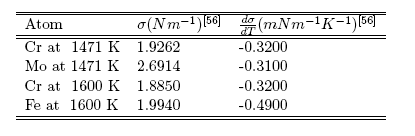The relations between the temperature dependence of surface tension and atomic volume have been used to get the atomic volumes required for the computation of surface concentration and surface tension, and these are given as [35, 56]: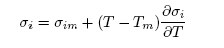(25)
and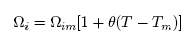(26)
where θ is the thermal coeﬃcient of expansion, Ωim, σim are the atomic volume and surface tension of the alloy components at their melting temperature Tm and T is the working temperature in Kelvins. The values of ∂σi/∂T and θ for the pure components of the alloys Cr, Mo and Fe were obtained from . S and Si for each atomic species of the alloy systems were computed using Eq. (23).
Lack of suﬃcient experimental data on surface properties for the two liquid alloys at the working temperatures justify the interest that can be given to the theoretical investigations. In particular, the concentration dependent data of surface tension (σ) for segregating liquid alloys are scarce . Fig. 10 shows the results of the computed values of surface concentration of Cr as a function of bulk concentration for the two liquid alloys. The structural analysis shows that as bulk concentration of Cr in Cr-Mo liquid alloys increases, the surface concentration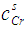remain constant at about 0.11% up till about 40% bulk concentration when it begins to rise sightly with increasing bulk concentration. At 96.5% and 98.9% bulk concentrations, the surface concentrations are 17.56% and 53.42%, respectively. This is an indication that in the alloy there are more atoms of component with bigger atomic size (i.e. Mo-atoms with atomic size of 9.38 , compared to Cr with 7.23 ) at the surface. Hence, at the surface of Cr-Mo, more Mo-atoms segregate at the surface in preference to Cr-atoms up to around 98.9% bulk concentration.
On the other hand, the calculated values of surface concentration,, in Cr-Fe liquid alloys (Fig. 10) suggest the segregation of Cr-atoms to the surface as the bulk concentration increases. The rate at which Cr- atoms segregates to the surface is nearly proportional to the rate at which it segregates to the bulk surface with the surface isotherm curve for Cr- Fe liquid alloys almost close to ideal mixture behaviour. This implies that Cr-atoms with lower value of surface tension with respect to Fe-atoms tend to segregate to the surface, while the Fe-atoms with higher surface tension value prefer to remain in the bulk in the molten alloy. A closer look at the surface isotherm curve also reveals that Cr-Fe liquid alloy exhibits a slight positive to negative deviations with respect to the ideality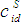. It may be point out here that when there is a signiﬁcant diﬀerence in surface tension values between the solvent and solute, the mixing shows strong segregation of the component with lower surface tension at the surface and the other in the bulk [6, 60].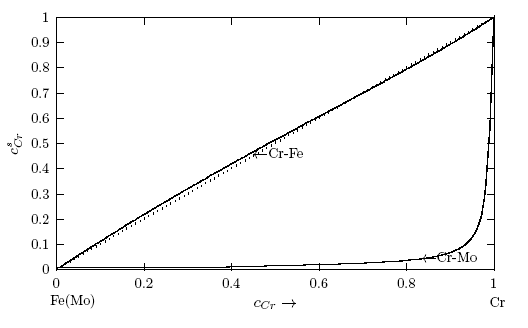Figure 10. Computed values of the surface concentrations of Cr in Cr-Mo and Cr-Fe liquid alloys at 1471 K and 1600 K, respectively. The curves were computed via Eq. (22). The dots denote the ideal values of the surface concentration,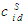computed from: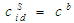. cCr is the bulk concentration of Cr in the alloys
Comparison of the two surface isotherm curves describing the surface segregation reveals that the rate at which Cr-atoms segregate at the surface of Cr-Fe liquid alloys is more than the rate at which Cr-atoms segregate at the surface in Cr-Mo liquid alloys for all bulk concentrations. For instance, at the same bulk concentration when cCr is equal to 40%, the surface is enriched with about 0.11% of Cr-atoms in Cr-Mo whereas about 42% of Cr- atoms segregate at the surface of Cr-Fe alloys. The enrichment of the surface of Cr-Fe alloys with Cr-atoms could be due to the fact that there exists a higher tendency for unlike atoms to pair as nearest neighbours in Cr-Fe alloys, than in Cr-Mo alloys which shows a higher degree of segregation as earlier observed for Scc(0) and α1. On the contrary, with very comparable values of the surface tension of the metals as noticed in Cr-Fe liquid alloy, the surface concentrations do not diﬀer much from the bulk compositions.
The surface tensions of the liquid alloys were computed by putting the surface concentration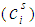values, W, surface coordination fractions (p and q), mean atomic surface area (S) and surface tension data of pure components (σA and σB) into Eq. (22). Surface tension data of pure Cr, Mo and Fe metals were taken from . The surface tension isotherms of Cr-Mo and Cr-Fe liquid alloys exhibit negative deviations from corresponding ideal mixture isotherms as shown in Figs. 11 and 12, respectively.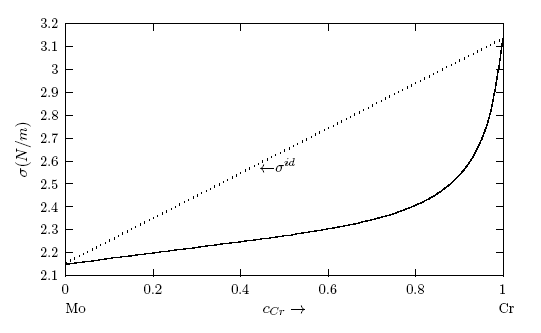Figure 11. Computed values of the surface tension, σ for Cr-Mo liquid alloys at 1471 K, computed via Eq. (22). The dots labelled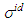denote the ideal values of the surface tension obtained from the relation: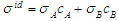. cCr is the bulk concentration of Cr in the alloy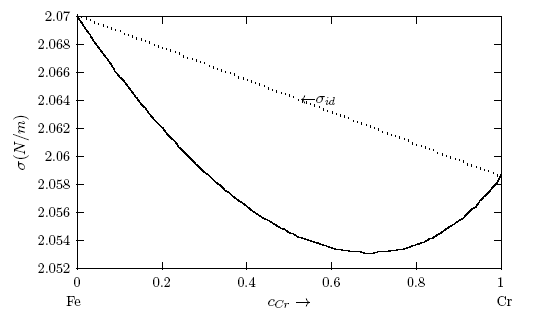Figure 12. Computed values of the surface tension, σ for Cr-Fe liquid alloys at 1600 K, computed via Eq.(22). The dots labelled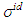denote the ideal values of the surface tension obtained from the relation: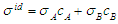. cCr is the bulk concentration of Cr in the alloy
The result shows that surface tension of Cr-Mo liquid alloy increases with increase in the bulk concentration of metal with lower surface tension (i.e. Cr-atoms) (Fig. 11). Whereas, the surface tension of Cr-Fe liquid alloy initially decreases with increasing bulk concentration to about 70% bulk concentration and then rises as seen in Fig. 12. The negative deviation between calculated surface tension isotherms of both systems and ideal values of the surface tensions substantiates earlier claim that the two liquid alloys are segregating systems. This submission remains consistent with the positive deviations of their bulk properties from the Raoult’s law [60, 61]. However, due to lack of experimental data at the investigation temperatures, it was impossible to compare the calculated surface tension values with experimental values.

### 4. Concluding Remarks

The theoretical study shows positive deviation in the mixing properties from the ideal mixture behaviour indicating segregation in Cr-Mo and Cr-Fe liquid alloys. The degree of segregation is more in Cr-Mo alloy at 1471 K than in Cr-Fe system at 1600 K as evident in their investigated bulk properties. It was pointed out that when there is a signiﬁcant diﬀerence in surface tension values between the solvent and solute, the mixing shows strong segregation of the component with lower surface tension at the surface and the other in the bulk. The surface tension study also reveals that addition of Cr-atoms leads to increase in surface tension of Cr-Mo and Cr-Fe liquid alloys across bulk concentration expect for bulk concentration < 70% in Cr-Fe, where σ decreases with increasing bulk concentrations. The decrease in σ of Cr- Fe alloys for bulk concentration in the range of 0 ≤ cCr ≤< 0.7 indicates that more atoms of components with bigger atomic size (i.e. Cr-atoms) segregates at the surface in preference to Fe-atoms. In addition, the rates at which Cr-atoms segregate at the surface of Cr-Fe liquid alloys is more than the rate at which Cr-atoms segregate at the surface in Cr-Mo liquid alloys for all bulk concentrations. This segregation of the components may result from the diﬀerences in atomic size, surface tension and electron conﬁguration between the components of an alloy. The possible roles of the entropic and enthalpic eﬀects on the phase-segregation observed in the two liquid alloys have been explained in terms of the bulk and surface properties.

### References

  R. Novakovic and J. Brillo, J. Molecul. liq., 200 (2014) 153-159.  C. Costa, S. Delsante, G. Borzone, D. Zivkovic, R. Novakovic, J. Chem. Thermodyn., 69 (2014) 73-84  Z. Grzesik, Z. Jurasz, K. Adamaszek, S. Mrowec, High Temp. Mater, Process, 31(6) (2012) 775-779.  C. Fazio, A. Alamo, A. Almazouzi, S. De Grandis, D. Gomez-Briceno, J. Henry, L. Malerba, M. Rieth, J. Nucl. Mater. 392 (2009) 316-323  B. Zheng, Y. Zhou, J.E. Smugerresky, E.J. Lavernia, Metall. Mater. Trans. A 40 (2009) 1235-1245.  R. Novakovic, J. Phys.: Condens. Matter 23 (2011) 235107 (8pp).  P. H. Mayrhofer, H. Willmann, and A. E. Reiter, Surf. and Coatings Techn., 202 (2008) 4935-4938.  P. H. Mayrhofer et al., Progress in Materials Science 51 (2006) 1032- 1114.  P. Hones, R. Sanjins, and F. Lvy, Thin Solid Films 332 (1998) 240-246.  B. Gu et al., Surf. and Coatings Technn. 202 (2008) 2189-2193.  L. Zhou, H. David, and H. M. Paul, J. Phys. D: Appl. Phys. 46 (2013) 365301.  A. Magnli, Acta Crystallographica 6 (1953) 495.  G. Gassner et al., Surf. and Coatings Techn. 201 (2006) 3335-3341.  K.T. Jacob, B.V. Kumar, Z. Metallkd. 77 (1986) 207-211.  M. Laﬃtte, O. Kubaschewski, Trans. Faraday Soc. 57 (1961) 932.  O. Kubaschewski, T.G. Chart, J. Inst. Met. 93 (1965) 329-338.  D.S. Dickson, J.R. Myers, M.J. Pool, R.K. Saxer, Trans. Metall. AIME 245 (1969) 175-177  S. Hertzman, B. Sundman, Calphad 6 (1982) 67-80.  J.O. Andersson, TRITA- MAC-0207, KTH, 1982.  Y.Y. Chuang, J.C. Lin, Y.A. Chang, Calphad 11 (1987) 57-72.  J.C. Lin, Y.Y. Chuang, K.C. Hsieh, Y.A. Chang, Calphad 11 (1987) 73-81.  R. Hultgren, P.D. Desai, D.T. Hawkins, M. Gleiser, K.K. Kelly, Selected Values of the Thermodynamic Properties of binary Alloys, American Society of Metals, Ohio, 1973.  J.O. Andersson, B. Sundman, Calphad 11 (1987) 83-92.  B.-J. Lee, CALPHAD 17 (1993) 251-268.  J. Vretl, J. Houserov, M. ob, J. Min. Metall. Sect. B 38 (2002) 205-211.  J.-C. Lin, Y.-Y. Chuang, K.-C. Hsieh, Y.A. Chang, Calphad 11 (1987) 73-81  W. Xiong, M. Selleby, Q. Chen, J. Odqvist, Y. Du, Crit. Rev. Solid State Mater. Sci. 35 (2010) 125-152.  W. Xiong, P. Hedstrom, M. Selleby, J. Odqvist, M. Thuvander, Q.Chen, Calphad 35 (2011) 355-366.  R.M. Fisher, E.J. Dulis, K.G. Carroll, Trans. Am. Inst. Min. Metall. Pet. Eng. 197 (1953) 690-695.  C.X. Quintela, B. Rodrguez-Gonzlez, and F. Rivadulla, Appl. Phys. Lett. 104 (2014) 022103-5.  S. Swaminathan, K.T. Jacob, Bull. Alloy Phase Diagrams 10 (1989) 329-331.  J.P. Neumann, Bull. Alloy Phase Diagrams 10 (1989) 524-524.  K. Mills and Y.C. Su, Int. Mater. Rev., 51(6) (2006) 329-351.  L. Pauling, Nature of the Chemical Bonding, Cornell University Press, Ithaca, NY. 1960.  T. Iida, and R.I.L. Guthrie, The Physical Properties of Liquid Metals, Clarendon Press, Oxford, 1993.  R.N. Singh, F. Sommer, Rep. Prog. Phys. 60 (1997) 57-150.  Y.A. Odusote, L.A. Hussain, O.E. Awe, J. Non-Cryst. Solids 353 (2007) 1167-1171.  R.N. Singh, N.H. March, in: J.H. Westbrook, R.L. Fleischer (Eds.), Intermetallic Compounds, Principles and Practice, Vol. 1, Wiley, New York. 1995, p.661-686.  R. Novakovic, M.L. Muolo, and A. Passerone, Surf. Sci., 549 (2004) 281-293.  R.N. Singh and F. Sommer, Z. fur Metallk. 83(7) (1992) 533-540.  J.M. Cowley, Phys. Rev. 77 (1950) 669-675.  R.N. Singh and F. Sommer, Phys. Chem. Liq., 36 (1998) 17-28.  S.M. Osman, R.N. Singh, Phys. Rev. E. 51 (1995) 332.  R.N. Singh, F. Sommer, J. Phys. Condens. Mat. 4 (1992) 5345-5358.  R.N. Singh, F. Sommer, Z. Metallkd. 83 (1992) 533-540.  L.C. Prasad, R.N. Singh, V.N. Singh and G.P. Singh, J. Phys. Chem. B, 102 (1998) 921-926.  L.C. Prasad, R.N. Singh and G.P. Singh, Phys. Chem. Liq., 27(3) (1994) 179-185.  E.A. Guggehheim, Mixtures, Oxford University Press, London, 1952.  R. N. Singh, Can. J. Phys. 65 (1987) 309-325.  I. Egry, E. Ricci, R. Novakovic, S. Ozawa, Adv. Colloid Interface Sci., 159 (2010) 198-212.  E. A. Moelwyn-Hughes, Physical chemistry, Pergamon Press, Oxford, 1961, p19.  J. Brillo, R. Brooks, I. Egry, P. Quested., Int. J. Mat. Res. (formerly Z. Metallkd.) 98 (2007) 6.  I. Budai, M. Z. Benk o, G. Kaptay, Materials Science Forum Vol. 473- 474 (2005) 309.  I. Budai, M. Z. Benk o, G. Kaptay, Materials Science Forum Vol. 537- 538 (2007) 489.  T. Iida, and R. I. L. Guthrie,, The Physical Properties of Liquid Metals, Clarendon Press, Oxford, 1993.  Smithell’s Metals Reference Book, in: W.F. Gale, T.C. Totemeir (Eds.), 8th Edition, Elsevier Butterworth-Heinemann, Oxford, 2004.  J. Brillo, I. Egry, T. Matsushita, Z. Metallkd. 97 (2006) 28.  Y.A. Odusote, J. Non-crystal. Solids, 402 (2014) 96-100.  C.N. Singman, J. Chem. Education, 61(2) (1984) 137-142.  R. Novakovic, T. Tanaka, M.L. Muolo, J. Lee, and A. Passerone, Surf. Sci., 591 (2005) 56-69.  T. Tanaka, K. Hack, S. Hara, Mater. Research Sci. Bull.(1999) 45.